# Cooker

A current of 2A passes through the immersion cooker at a voltage of 230V. What work do the electric field forces in 2 minutes?

Correct result:

W =  55200 J

#### Solution: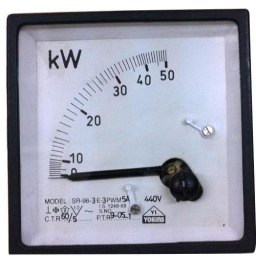We would be pleased if you find an error in the word problem, spelling mistakes, or inaccuracies and send it to us. Thank you!Tips to related online calculators
Do you want to convert time units like minutes to seconds?

## Next similar math problems:

• Current in the conductorCalculate the current in the conductor (in mA) if it is connected to a 4.5 V voltage source and its resistance is 20 (ohm).
• The bulbWhat is the resistance of a filament lamp when it is connected to a 9V battery and has a current of 120 mA?
• The bulbThe bulb has a reading of 6V-0.05 A, what current flows through the bulb if we connect it to a cell with a voltage of 12V?
• Coils of transformerThe primary coil of the transformer has 1100 turns and is connected to a voltage of 220V. How many turns does the secondary coil have when the voltage on it is 55 V? Determine the transformation ratio and decide what kind of transformation is it.
• Coils of transformerThe primary coil of the transformer has 400 turns, a current of 1.5 A passes through it and is connected to a voltage of 220 V. For the secondary coil, find the voltage, current, and a number of turns if the transformation ratio k = 0.1.
• TransformerTransformer - U1 = 230 V, N1 = 300, N2 = 1,200, I1 = 4 A. Calculate the transformation ratio, voltage and current in the secondary coil.
• Electric workCalculate the work done by the electric forces passing the current of 0.2 A through the bulb in 10 minutes if the bulb is connected to a 230 V power supply.
• Two resistorsTwo resistors 20 Ω and 60 Ω are connected in series and an external voltage of 400 V is connected to them. What are the electrical voltages on the respective resistors? Please comment!
• Lowest voltage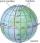Three resistors with resistors R1 = 10 kΩ, R2 = 20 kΩ, R3 = 30 kΩ are connected in series and an external voltage U = 30 V is connected to them. On which resistor is the lowest voltage?
• Mains voltageHow much electric current flows through the appliance with a resistance of 40kΩ, which is connected to the mains voltage (230 V)?
• Copper Cu wireCopper wire with a diameter of 1 mm and a weight of 350 g is wound on a spool. Calculate its length if the copper density is p = 8.9 g/cm cubic.
• Closed circuitIn a closed circuit, there is a voltage source with U1 = 12 V and with an internal resistance R1 = 0.2 Ω. The external resistance is R2 = 19.8 Ω. Determine the electric current and terminal voltage.
• Energy consumption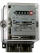The device is connected to 230V and draws 3.5A current. Power consumption is 1932kJ. How many minutes has this device been in operation?
• The copper wireThe copper wire bundle with a diameter of 2.8mm has a weight of 5kg. How many meters of wire is bundled if 1m3 of copper weighs 8930kg?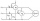Two wattmeters are connected to measuring power in a 3 phase balanced load. Determine the total power and power factor if the two wattmeters read 1000 watts each (1) both positive and (2) second reading is negative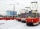If the Lewis family used 648 kilowatt-hours of electricity in 12 days, how many kilowatts hours should they use in 24 days at the same rate of usage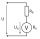We have a voltmeter which in the original set measures voltage to 10V. Calculate the size of the ballast resistor for this voltmeter, if we want to measure the voltage up to 50V. Voltmeter's internal resistance is 2 kiloohm/Volt.